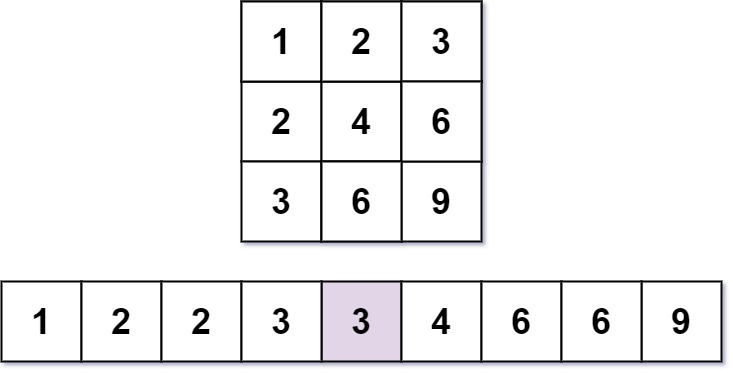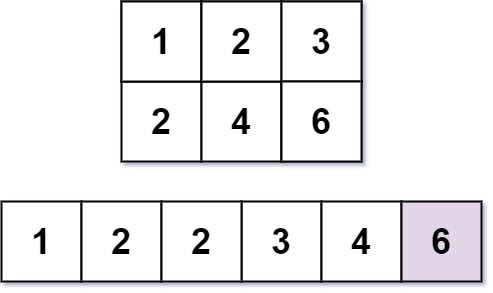668. Kth Smallest Number in Multiplication Table
Hard
2K
52

Nearly everyone has used the Multiplication Table. The multiplication table of size `m x n` is an integer matrix `mat` where `mat[i][j] == i * j` (1-indexed).

Given three integers `m`, `n`, and `k`, return the `kth` smallest element in the `m x n` multiplication table.

Example 1:```Input: m = 3, n = 3, k = 5
Output: 3
Explanation: The 5th smallest number is 3.
```

Example 2:```Input: m = 2, n = 3, k = 6
Output: 6
Explanation: The 6th smallest number is 6.
```

Constraints:

• `1 <= m, n <= 3 * 104`
• `1 <= k <= m * n`
Accepted
56.5K
Submissions
110K
Acceptance Rate
51.4%

Seen this question in a real interview before?
1/4
Yes
No

Discussion (0)

Related Topics